# The Art of Electronics - THERMAL STABILITY OF CLASS B PUSH-PULL FOLLOWER AMP (pg.93)

#### vol_

Joined Dec 2, 2015
93
I'm working 5 hrs on this and still i dont get a clue at all.. Everything i tried is not working and i tried too much things in different ways, but i dont think these will help anyone.

This is the schematic of the push-pull follower amplifier that I want to calculate its thermal stability (I want to verify the thermal stability actually):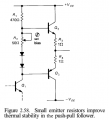And this is the text where the authors analyze the thermal stability: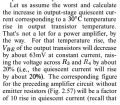And my questions are:

1) How the voltage across R3 and R4 is raised by 20%???

2) In quiescent conditions the base current for Q2 will be: 0,6V / 470 ohm = 1 mA.
So the emitter current, assuming β=100, will be: 1mA * 100 = 0,1 A
From this we get an intrinsic resistance for Q2 with value: re = 25 / Ic =25 / 0,1 = 250 ohm, which is much bigger than the R3 and R4 values.

In which way these little resistor make such a big difference and shouldn't the authors take them into account in their calculations? Or they do take them?

#### dannyf

Joined Sep 13, 2015
2,197
If the differential between the two bases is maintained a constant, any decline in vbe, from the transistor heating up, will be dropped on the emitter resistors, causing the idle current to go up, dissipation to go up, and the temperature to go up, ....

#### crutschow

Joined Mar 14, 2008
31,538
1) The Vbe changes by about -2mV/°C. This gives the -63mV for a 30°C change the author noted.
This causes a change in the emitter voltage across R3 and R4 which changes the bias current.
To calculate the percent change in bias current, you need the bias current value, which you don't show.

2)Your calculations for the bias current are not correct.
Why do you think it's 0.6V / 470?
To calculate the output bias current you need to know the setting of R2, the characteristics of the diodes and how much bias current Q1 is conducting.

#### Jony130

Joined Feb 17, 2009
5,439
1) How the voltage across R3 and R4 is raised by 20%???
Let us assume that IcQ2 =IcQ3 = 0.1A so we have 0.2 voltage drop across R3+R4. Now let us assume VbeQ1 =VbeQ2 = 0.61V at Ic = 0.1A, the voltage difference between transistors bases is Vb2 - Vb3 = 0.2V + 2*0.61V = 1.42V and this difference is constant (does not depend temperature).
As temperature rise by 30° the Vbe will drop about -2.1mV/°C, so we have 30*-2.1mV = -63mV. Therefore the new Vbe value is 0.547V and new voltage across R3 + R4 is 1.42V - 2*0.547V = 0.32V. This give us the new Icq value for a output stage Icq = 0.32V/2Ω = 160mA.
And voltage rise from 0.2V to 0.32V. Or in simple terms, if Vbe decrease by 63mV the voltage across emitter resistors must rise by this 63mV (because Vb2 -Vb3 is constant). From 0.1V to 0.163V.
But now if we include this two diodes (diode voltage will also drop about -2mV/°C) and assume that they are tracking Q2 and Q3 temperature (diodes are thermally coupled to the output transistors). We have : Vb1 - Vb2 = 1.42V - 63mV = 1.36V. And voltage drop across emitter resistors is 1.36V - 2*0.547V= 0.266V. This means that the output current increases from 100mA to 133mA about 33%.
So, I don't know from where they get this 20%. All this means I must have made a slip somewhere but I do not see it.
In quiescent conditions the base current for Q2 will be: 0,6V / 470 ohm = 1 mA.
So the emitter current, assuming β=100, will be: 1mA * 100 = 0,1 A
How can this be true ? Can you explain?
intrinsic resistance
re - is a AC signal resistance re = Δvbe/Δie (dynamic resistance), so, for DC re = 0Ω.

Last edited:
•vol_

#### RBR1317

Joined Nov 13, 2010
706
In the previous paragraph the author verbally changed the circuit from the image posted here. The 470Ω resistor does not affect the biasing calculations, only the three series diodes do. In parallel with these 3 diodes are R3 & R4 and the Q2 & Q3 base-emitter junctions, therefore the equivalent of one diode voltage drop appears across R3+R4.

When the temperature of Q2 & Q3 rises and the Vbe drops by 10%, the voltage across each emitter resistor must rise by the same 10% (because the voltage across the 3 diodes did not change) so the total voltage across the R3+R4 resistance increased by 20% and the quiescent current will rise by about 20%.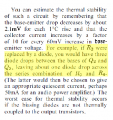Last edited:
•vol_ and Jony130

#### vol_

Joined Dec 2, 2015
93
1) The Vbe changes by about -2mV/°C. This gives the -63mV for a 30°C change the author noted.
This causes a change in the emitter voltage across R3 and R4 which changes the bias current.
To calculate the percent change in bias current, you need the bias current value, which you don't show.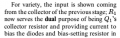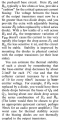The previous and the above is all the information we have. There is no other clue that will help us find the bias current.

2)Your calculations for the bias current are not correct.
Why do you think it's 0.6V / 470?
To calculate the output bias current you need to know the setting of R2, the characteristics of the diodes and how much bias current Q1 is conducting.
The diodes are in forward conduction Holding Q2's base two diode drops above the input signal and Q3's base one diode drop above the input signal. The input signal is ground referenced. Thats why i think that the bias current for Q2 will be 0,12mA.

I'm really tired right now. I hope i explained my self good.. I can barely see..

#### Jony130

Joined Feb 17, 2009
5,439
The diodes are in forward conduction Holding Q2's base two diode drops above the input signal and Q3's base one diode drop above the input signal. The input signal is ground referenced. Thats why i think that the bias current for Q2 will be 0,12mA.
But this is not the case here. And the input signal is not ground referenced. And Q1 provide a "current steering" and Q1 emitter is usually connected to Vee , and to properly bias this circuit we are forced to use a negative feedback

#### vol_

Joined Dec 2, 2015
93
I'm sorry about not I didnt give all the info about the problem.. Next time that I'll be studying 12 hrs i will expect the next day to create a thread or post a reply..

...therefore the equivalent of one diode voltage drop appears across R3+R4.
When the temperature of Q2 & Q3 rises and the Vbe drops by 10%, the voltage across each emitter resistor must rise by the same 10% (because the voltage across the 3 diodes did not change) so the total voltage across the R3+R4 resistance increased by 20% and the quiescent current will rise by about 20%.
So we dont even use the fact that a diode voltage drop appears across R3+R4. It is just the change of Vbe by the initial Vbe: 0.063V / 0.6V x 100%=10,5%. This times 2 for the two transistors (20%) will be the change of voltage across R3+R4 independent of the voltage drop across them. Tell me if I am wrong.

Cheers!

#### RBR1317

Joined Nov 13, 2010
706
Knowing the actual voltage across R3+R4 will give the actual Q-point current, but for finding the thermal stability one is only interested in finding the relative change in the current.

#### vol_

Joined Dec 2, 2015
93
For this circuit (figure 2.58), but with a diode instead a resistor in the biasing base voltage, what i can not understand is in what operating stages Q2 and Q3 are.
The base of Q2 is 3 diodes voltage drop above signal (for positive swings) and the base of Q3 is at the same level with the signal for (negative swings). Is this right? Also, we will have a diode voltage drop across R3+R4 (0,6V).
Is the R3+R4 voltage drop summed up with Q2's emitter voltage and subtracted from Q3's emitter voltage?

#### Jony130

Joined Feb 17, 2009
5,439
For this circuit (figure 2.58), but with a diode instead a resistor in the biasing base voltage, what i can not understand is in what operating stages Q2 and Q3 are.
The base of Q2 is 3 diodes voltage drop above signal (for positive swings) and the base of Q3 is at the same level with the signal for (negative swings). Is this right? Also, we will have a diode voltage drop across R3+R4 (0,6V).
Is the R3+R4 voltage drop summed up with Q2's emitter voltage and subtracted from Q3's emitter voltage?
For this circuit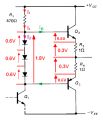Without knowing the rest of the circuit, all we can tell is that R1 current will split (divide) into 3 diodes current and into Ib current.
But if we look closely you should notice that thous 3 diodes are connect in parallel with Q2+R3+R4+Q3.
And thank to this we can write a KVL around the loop
3*0.6V = Vbe - VR3 - VR4 - Veb and from this VR3+VR4 = 3*0.6V - (Vbe+Veb) = 0.6V
So, the Q2,Q3 quiescent current is around 0.6V/2Ω = 0.3A
Also try to read chapter 4.72 (figure 4.72)

#### vol_

Joined Dec 2, 2015
93
Lets assume Vin=-1V.
For Q2 we will have Ve2=0,2V < Vb2=0,8V < Vc2=15V.
And for Q3: Ve3=-1,6V < Vb3=-1V > Vc3=-15V.
This is wrong. But I dont know what I m doing wrong. If I knew...

And here i dont include R3+R4 voltage drop impact on Ve2, Ve3. If i did i would write Ve2=0,8V. or Ve2=-0,4V.. Ohh I m tired again..

#### Jony130

Joined Feb 17, 2009
5,439

#### vol_

Joined Dec 2, 2015
93
Vin ?? Where??
At Q1's collector, lets assume a Vin=-1V..

I am actually trying to understand how Q2 and Q3 operate during a cycle of signal. I know that between +0,6V and -0,6V they operate simultaneously, but o cant figure it out..

#### Jony130

Joined Feb 17, 2009
5,439
First of all Q1 collector do not act as a voltage source.
I am actually trying to understand how Q2 and Q3 operate during a cycle of signal. I know that between +0,6V and -0,6V they operate simultaneously, but o cant figure it out..
Without including the load resistance you won't see anything.
For positive voltage load resistance will sink the current from Q2 so less current is left for Q3.
And this is why Q2 turns on "more" and Q3 conducts "less".

Last edited:

#### vol_

Joined Dec 2, 2015
93
And this is why Q2 turns on "more" and Q3 conducts "less".
Oh that 'more' or 'less' helped me a lot.. I will exam the clash b push pull amp that you described in a thread tomorrow..

#### vol_

Joined Dec 2, 2015
93Hello again,

Here I still cant get why T2 is cut off. The b-e junction is not reverse biased (Vb2=+1,4V and Ve2=+2V) and we have Ve2=2V > Vb2=1,4V > Vc2=-10V. So what i get for the pnp is that is forward active..

Hi Jony,
How can you know T2 is OFF?
I can't explain it. Veb = 0.7V and Vec = 12V and therefore I think it will conduct.
But in this circuit Vbe = -0.6V. So knowing that Vbe is equal to -0.6V.
Can you tell me in which state BJT is?

#### dannyf

Joined Sep 13, 2015
2,197
You cannot. The base current is in-determinant and the voltage on the emitter is incalculable.

The circuit is really just a folliwer. With feedback, you can not determine what the output voltage is.

#### Jony130

Joined Feb 17, 2009
5,439
The answer is not so simple. And this is why I assume that T2 is off. But let me try to explain this.
First notice that as long as the load is disconnected we have Ic1 = Ic2. And we can say that T1 and T2 are connected in series.
Now if we connect the load we have :
During the positive half cycle the voltage at the load increases, this means that Ic1 and Vbe1 also must increases at the same time Vbe2 and Ic2 decreases. Because T1 conducts more current the less current is left for T2 (Ic2 = Ic1 - IL)
Or the voltage difference between transistor bases is constant and equal to 2Vbe (D1 +D2). Therefore we have 2Vbe = Vbe1 + Vbe2 and if Vbe1 increases this will automatically reduces Vbe2 and Ic2 (because Vbe1 + Vbe2 mus be equal to 2Vbe).
And in real life T2 will cut-off if ILoad is larger than 2 times bias current.

#### vol_

Joined Dec 2, 2015
93
The answer is not so simple. And this is why I assume that T2 is off. But let me try to explain this.
First notice that as long as the load is disconnected we have Ic1 = Ic2. And we can say that T1 and T2 are connected in series.
Now if we connect the load we have :
During the positive half cycle the voltage at the load increases, this means that Ic1 and Vbe1 also must increases at the same time Vbe2 and Ic2 decreases. Because T1 conducts more current the less current is left for T2 (Ic2 = Ic1 - IL)
Or the voltage difference between transistor bases is constant and equal to 2Vbe (D1 +D2). Therefore we have 2Vbe = Vbe1 + Vbe2 and if Vbe1 increases this will automatically reduces Vbe2 and Ic2 (because Vbe1 + Vbe2 mus be equal to 2Vbe).
And in real life T2 will cut-off if ILoad is larger than 2 times bias current.
Thanks mate! I was aware, already, of your answer from your five years older posts. I think thats all for now. See you at the differential amps, although i wish i wont have so vast questions after the brilliant answers i got from you and the other guys the previous days. Keep on sharing!
Cheers!